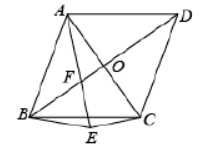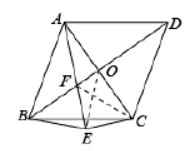$\text{A.}$ $4 \sqrt{6}$ $\text{B.}$ $4 \sqrt{3}$ $\text{C.}$ $4 \sqrt{2}$ $\text{D.}$ $4$

B

#### 解析：$\because$ 菱形 $A B C D, A C=2$,
$$\therefore A O=O C=1, A C \perp B D$$

\begin{aligned} & \therefore O E=O C=1, \angle E O C=2 \angle C A E \\ & \text { 又 } \angle E B F=2 \angle C A E \\ & \therefore \angle E B F=\angle E O C \\ & \angle B F E=\angle A F O, \\ & \therefore \angle B F E+\angle F A O=90^{\circ} \\ & \text { 又 } \angle F A O+\angle O C E=90^{\circ} \\ & \therefore \angle B F E=\angle O C E \\ & \text { 在 } \triangle B F E \text { 和 } \triangle O C E \text { 中, } \\ & \angle E B F=\angle E O C \\ & B F=O C \\ & \angle B F E=\angle O C E \\ & \therefore \triangle B F E \cong \triangle O C E(A S A) \\ & \therefore F E=C E \end{aligned}

\begin{aligned} & F E=E C, \angle F E C=90^{\circ} \\ & \therefore F E=E C=\sqrt{\frac{x^2+1}{2}} \end{aligned}

\begin{aligned} & \therefore\left(\sqrt{x^2+1}+\sqrt{\frac{x^2+1}{2}}\right)^2+\left(\frac{\sqrt{x^2+1}}{2}\right)^2=2^2 \\ & \therefore x^2=3-2 \sqrt{2}=(\sqrt{2}-1)^2 \end{aligned}
\begin{aligned} & \therefore x=\sqrt{2}-1, x_2=1-\sqrt{2} \text { (舍去) } \\ & \therefore O B=\sqrt{2} \\ & \therefore A B=\sqrt{O A^2+O B^2}=\sqrt{3} \end{aligned}
$\therefore$ 菱形 $A B C D$ 的周长为 $4 A B=4 \sqrt{3}$,